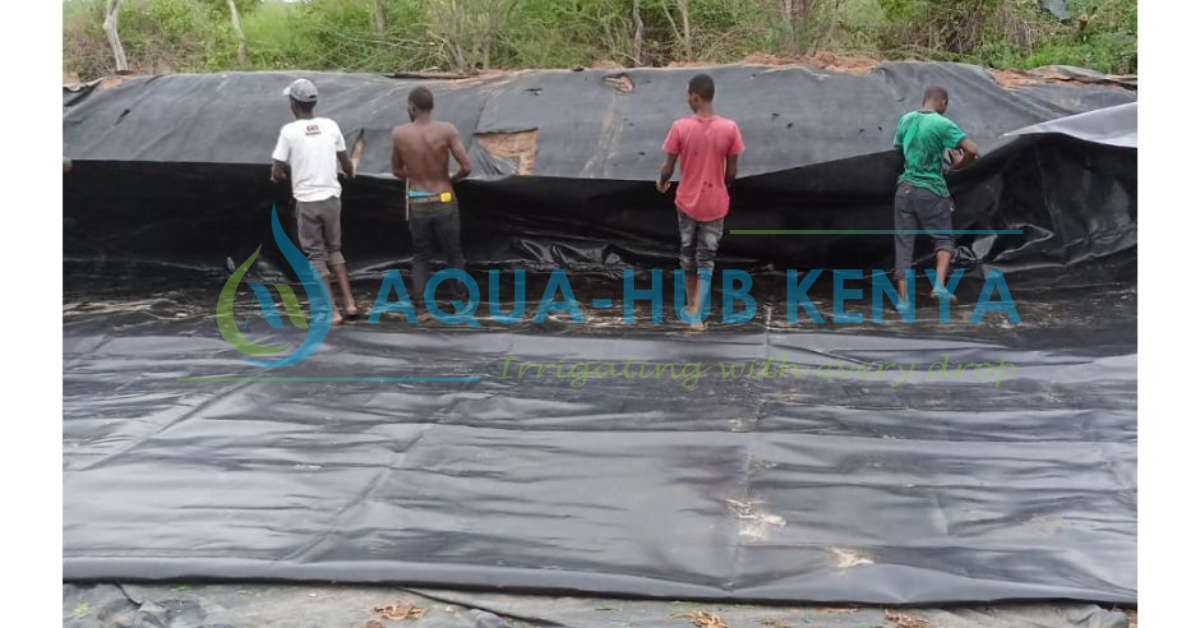Dam liner CalculatorOur dam liner calculator makes it easy to determine the size of liner you need for your pond or dam. The size of the dam liner needed for the dam liner construction can be easily determined using the dam liner calculator. The formula of calculating the dam liner size is (Length + (2 x Depth + 1)) by (Width + (2 x Depth + 1)). Determining the size of a dam liner required for dam lining or fishpond lining is a constant challenge for farmers. Using a straightforward calculator for dam liners, we can resolve this.

The final dam liner requirement is in square meters that we determine by multiplying the reservoir’s length, breadth, and depth by the dam liner calculator formula. The dam liner calculator is essentially the same for cylinder-shaped and rectangular reservoirs. It is easy to complete installation tasks with the right size, you get value for your money, and there is no extra, unused liner material lying around. No matter how thick the dam liner is, the liner material is the same size.

### What are the factors to consider when choosing a dam liner?

Some of the factors that influence liner thickness selection are reservoir size and surface roughness. The thicker the liner required to support the enormous water weight, the greater the capacity. The 0.5mm thick dam liner requires smooth surfaces (either dirt or cemented). Surfaces with murram or tiny stones require a 0.75mm thick liner, whereas those with larger stones or rocks require a 1mm or thicker liner. This is due to the fact that the thicker it gets, the more difficult it is to rip.

### How are dam liners calculated?

You may spend less money if you are aware of how much pond liner you need. Anything that is either too big or too little is a waste of money. Calculating how much material you’ll need requires precise measurements of your pond project. These will have to be taken into account while calculating liners. Your dimensions will be used to determine how much pond liner and protective underlay to purchase.

Dam liners provide you with greater creative flexibility when designing ponds since they give you additional design options. The further you deviate from the typical rectangular or circular pond design, the more measurement skills you’ll require. Never forget to increase the amount for a full overlay. Measurements must be taken in order to determine the quantity of dam liner required. The things you have to measure include;

1. Take the longest length.
3. Measure the depth of your pond at its deepest point.

Next, multiply one side (Length or Width) by two times the depth plus the amount of overlap (0.15m). The pond’s depth must be extended by two since the pond liner must go down one side and back up the other. The amount of overlay or overlap you should include in your estimate is totally dependent on how you want to install your pond liner. As a consequence, depending on the installation and edging method, adjust the amount of overlay.

### Calculating the size of the dam liner needed using the Dam liner Calculator

You must figure out how much liner material you’ll need in order for it to match your pond design. There is an easy method for figuring out the right liner sheeting size for common pond designs. The widest, longest, and deepest points in your pond’s design should all be measured in feet. The depth of the pond should be increased by two and one feet. This amount multiplied by this number times by the width of your pond gives you the required sheet size. The calculation is as follows: (Length + (2 x Depth + 1) by (Width + (2 x Depth + 1)

#### Calculation of Dam liner required for rectangular dam

Use the dam liner calculator formula to figure out how big a dam liner you’ll need:

Add overall length + twice the allowed depth plus a meter or more on either side for overlap to get the length (longer side).

For width, add overall length plus twice the maximum depth plus a meter or more on either side for overlap (short side).

For instance, assuming you have a pond measuring 15m long by 5m wide and 3m deep.

Dam liner required =?

Length = L+2*D+ (1+1)

Width = W+2*D+ (1+1)

Therefore, Length = 15 + (2*3) +2

= 15+6+2

Length = 23m

Width = 5 + (2*3) +2

= 5+6+2

Width = 13m

So dam liner required= 23m x 13m.

NB: Allow 2m for overlap to ensure the dam liner is securely fastened around the sides.

#### Calculation of Dam liner required for Cylindrical/Circular dam

Simply double the diameter of the tank by twice its height, adding a margin on either side for overlap, to get measurements for a cylindrical tank.

For instance, the diameter is 15m, the height is 4m, and the allowance for one side is 0.5m. The dam liner required will be:

Length = 15m + (3+3) + (0.5+0.5)

=15+6+1

= 22m

Since it’s a circular tank, the length equals the width, so width = 22m.

Therefore, Dam liner required = 15m by 15m = 484m2

### Where to buy dam liner in Kenya

High-end dam liners can be purchased from Aqua Hub. We are aware of how important a top-notch dam liner is for you to have for your project. As a result, we only offer dam liners that are of the highest caliber and designed to endure in a dam. Furthermore, you may be sure that you will receive the harvest you deserve because our dam liners do not poison fish. For additional information, to schedule a site visit, or to request a quote for your project, call us at NAIROBI: 0790719020 or ELDORET: 0759372241.